﻿ 惯性管型脉管内气体振荡制冷机理的分子动力学模拟

# 惯性管型脉管内气体振荡制冷机理的分子动力学模拟Molecular Dynamics Simulation of Gas Oscillation Refrigeration Mechanism in Inertia Tube Pulse Tube

Abstract: In this paper, the molecular dynamics simulation method is used to study the thermodynamic mechanism of the oscillation in the inertial tube (IT). The results show that the temperature, pressure and massflow phase of the inertia tube are delayed, the phase difference between the massflow and pressure wave is reduced, and the cooling efficiency of the pulse tube is improved. At the same time, the amplitude of massflow near the hot end decreases, while that near the cold end increases. In addition, the gas temperature in the IT is reduced. The simulation results are helpful to understand the thermodynamic mechanism of the internal oscillation of the IT.

1. 简介

2. 原理

2.1. 分子动力学模拟

${F}_{i}=-{\nabla }_{i}U=-\left(i\frac{\partial }{\partial {x}_{i}}+j\frac{\partial }{\partial {y}_{i}}+k\frac{\partial }{\partial {z}_{i}}\right)U$ (1)

${a}_{i}=\frac{{F}_{i}}{{m}_{i}}=\frac{\partial {v}_{i}}{\partial t}=\frac{{\partial }^{2}{r}_{i}}{\partial {t}^{2}}$ (2)

a为原子加速度，F为所受力，m为其质量，v和r为其速度和位置。而且

${F}_{i}=\underset{j\ne i}{\sum }{F}_{ij}=-\underset{j\ne i}{\sum }{\nabla }_{ij}{U}_{ij}$ (3)

${U}_{ij}={G}_{i}\underset{i=1}{\overset{N}{\sum }}{\rho }_{h,i}+\frac{1}{2}\underset{i=1}{\overset{N}{\sum }}\underset{i\ne i}{\sum }{\varphi }_{ij}\left({r}_{ij}\right)$ (4)

${\rho }_{h,i}=\underset{j=1,j\ne i}{\overset{N}{\sum }}{\rho }_{j}\left({r}_{ij}\right)$ (5)

${\rho }_{i}\left({r}_{ij}\right)=\underset{k=1}{\overset{2}{\sum }}{A}_{k}{\left({R}_{k}-{r}_{ij}\right)}^{3}H\left({R}_{k}-{r}_{ij}\right)$ (6)

${\varphi }_{ij}\left({r}_{ij}\right)=\frac{1}{4\pi {\epsilon }_{0}}\frac{Z\left(i\right)Z\left(j\right)}{{r}_{ij}}$ (7)

U为原子对势，G为嵌入能，表示原子i嵌入其他邻近原子在该处产生的电荷密度的能量， $\rho$ 为电荷密度， $\varphi$ 为原子间相互斥能。

${U}_{ij}=4\epsilon \left[{\left(\frac{\sigma }{{r}_{ij}}\right)}^{12}-{\left(\frac{\sigma }{{r}_{ij}}\right)}^{6}\right]$ (8)Table 1. L-J potential energy model parameters

${T}_{cal}=\frac{\underset{i=1}{\overset{N}{\sum }}{m}_{i}\left[{\left({v}_{i,x}-{v}_{ci,x}\right)}^{2}+{\left({v}_{i,y}-{v}_{ci,y}\right)}^{2}+{\left({v}_{i,z}-{v}_{ci,z}\right)}^{2}\right]}{3N{k}_{B}}$ (9)

${v}_{ci}=\frac{\underset{i=1}{\overset{N}{\sum }}{v}_{i}}{N}$ (10)

$p=\frac{NRT}{V}$ (11)

2.2. 分子动力学模型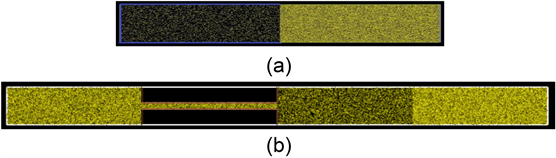Figure 1. (a) Basic pulse tube; (b) IT pulse tube

3. 仿真模拟结果与分析

3.1. 惯性管型脉管模拟结果及分析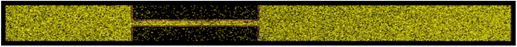Figure 2. IT pulse tube after operation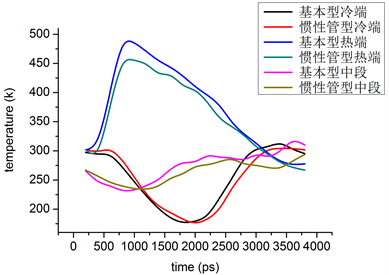Figure 3. Temperature changes with time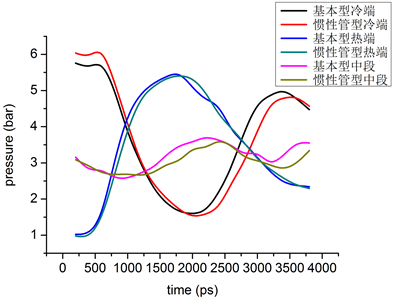Figure 4. Pressure changes with time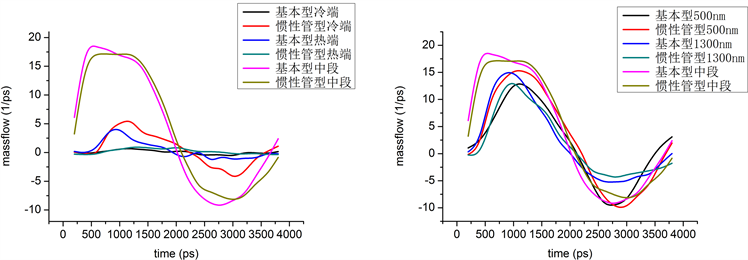Figure 5. Massflow changes with time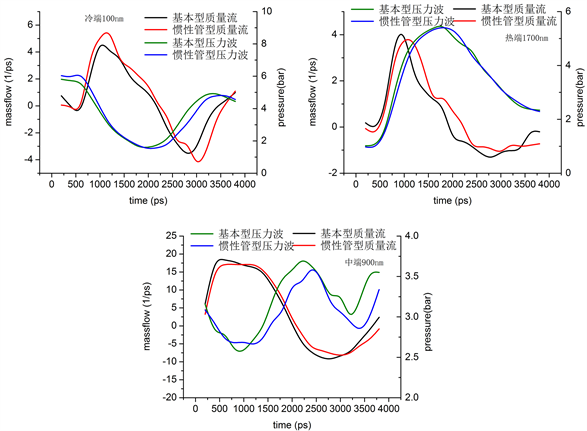Figure 6. Phase difference between massflow and pressure wave

3.2. 惯性管型脉管特点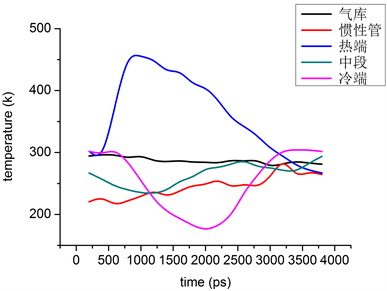Figure 7. Temperature changes with time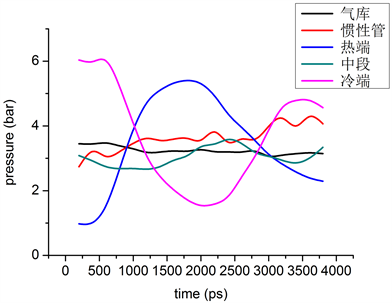Figure 8. Pressure changes with time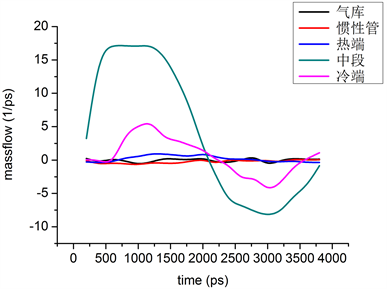Figure 9. Massflow changes with time

4. 结论

NOTES

*通讯作者。

 Radebaugh, R., Lewis, M.A., Luo, E.C., et al. (2006) Inertance Tube Optimization for Pulse Tube Refrigerators. Advances in Cryogenic Engineering, 51, 59-67.
https://doi.org/10.1063/1.2202401

 Luo, E., Radebaugh, R. and Lewis, M. (2004) Inertance Tube Models and Their Experimental Verification. AIP Conference Proceedings, 710, 1485-1492.
https://doi.org/10.1063/1.1774842

 Roach, P.R. and Kashani, A. (1997) Pulse Tube Coolers with an Inertance Tube: Theory, Modeling and Practice. In: Kittel, P., Ed., Advances in Cryogenics Engineering, Plenum Press, New York, 1895-1902.
https://doi.org/10.1007/978-1-4757-9047-4_239

 Dai, W., Hu, J. and Luo, E. (2006) Comparison of Two Different Ways of Using Inertance Tube in a Pulse Tube Cooler. Cryogenics, 46, 273-277.
https://doi.org/10.1016/j.cryogenics.2005.11.023

 胡剑英, 戴巍, 罗二仓, 等. 脉冲管制冷机调相机构的研究第二部分: 惯性管有无气库的研究[J]. 低温与超导, 2008, 36(9): 5-10.

 胡剑英. 液氮至液氢温区的热声驱动低温制冷机的研究[D]: [博士学位论文]. 北京: 中科院理化技术研究所, 2007.

 Schunk, L.O. (2004) Experimental Investigation and Modeling of Inertance Tubes. University of Wisconsin-Madison, Madison, WI.

 刘少帅, 张华, 张安阔, 等. 脉管制冷机单段和双段惯性管调相性能对比[J]. 制冷技术, 2016, 36(6): 10-15.

 Schunk, L.O., Pfotenhauer, J.M., Nellis, G.F., et al. (2004) Inertance Tube Optimization for kw-Class Pulse Tube. American Institute of Physics Conference Series, 710, 1269-1276.
https://doi.org/10.1063/1.1774814

 Alder, B.J. and Wainwright, T.E. (1957) Phase Transition for a Hard Sphere System. The Journal of Chemical Physical, 27, 1208-1209.
https://doi.org/10.1063/1.1743957

 Finnis, M.W. and Sinclair, J.E. (1984) A Simple Empirical N Body Potential for Transition Metals. Philosophical Magazine A, 50, 45-55.
https://doi.org/10.1080/01418618408244210

 Mendelev, M.I., Han, S., Srolovitz, D.J., Ackland, G.J. and Sun, D.Y. (2003) Development of New Interatomic Potentials Appropriate for Crystalline and Liquid Iron. Philosophical Magazine, 83, 3977-3994.
https://doi.org/10.1080/14786430310001613264

 Rappe, A.K., Casewit, C.J., Colwell, K.S., Goddard III, W.A. and Skiff, W.M. (1992) UFF, a Full Periodic Table Force Field for Molecular Mechanics and Molecular Dynamics Simulations. Journal of the American Chemical Society, 114, 10024-10035.
https://doi.org/10.1021/ja00051a040

 Kanao, K., Watanabe, N. and Kanazawa, Y. (1994) Aminiature Pulse Tube Refrigerator for Temperature below 100 K. Cryogenics, 34, 167-170.
https://doi.org/10.1016/S0011-2275(05)80035-9

 Fang, L., Deng, X. and Bauwens, L. (2003) Pulse Tube Refrigerator Analysis, Including Inertance Tube and Friction in the Regenerator. In: Ross R.G., Ed., Cryocooler 12, Springer, Boston, MA, 389-396.
https://doi.org/10.1007/0-306-47919-2_51

 Mikulin, E.I., Tarasov, A.A. and Shrebyonock, M.P. (1984) Low Temperature Expansion Pulse Tube. In: Fast, R.W., Ed., Advances in Cryogenic Engineering. Advances in Cryogenic Engineering, Vol. 29, Springer, Boston, MA, 629-637.
https://doi.org/10.1007/978-1-4613-9865-3_72

Top